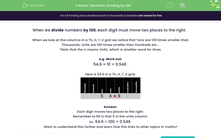# Practise Dividing Decimals by 100

In this worksheet, students divide numbers by 100 by shifting digits two places to the right in a grid.Key stage:  KS 2

Curriculum topic:   Number: Multiplication and Division

Curriculum subtopic:   Multiply/Divide Using Decimals

Popular topics:   Decimals worksheets, Place Value worksheets

Difficulty level:#### Worksheet Overview

When we divide numbers by 100, each digit must move two places to the right.

When we look at the columns in a Th, H, T, O grid we notice that tens are 100 times smaller than thousands, ones are 100 times smaller than hundreds etc...

e.g. Work out:

54.6 ÷ 100 = 0.546

Here is 54.6 in a place value grid.5 4 . 6

Each digit moves two places to the right.

Remember to fill in that 0 in the ones column.

So  54.6 ÷ 100 = 0.546

### What is EdPlace?

We're your National Curriculum aligned online education content provider helping each child succeed in English, maths and science from year 1 to GCSE. With an EdPlace account you’ll be able to track and measure progress, helping each child achieve their best. We build confidence and attainment by personalising each child’s learning at a level that suits them.

Get started